Home > AC > Chapter 5 > Lesson 5.1.5 > Problem5-50

5-50.
1. Multiply each of the following expressions. Show all of your work. Homework Help ✎

1. (x + 3)(4x + 5)

2. (−2x − 4)(3x + 4)

3. (3y − 8)(−x + y)

4. (y − 4)(3x + 5y − 2)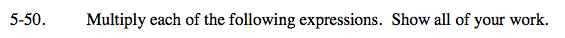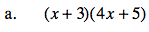Use a generic rectangle to multiply.

Put the products on the outside of the rectangle.

Use length times width to find the areas of the small rectangles.

Write the total area as a sum.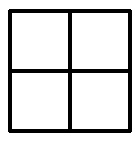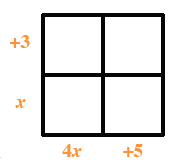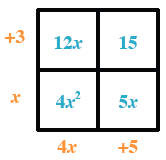4x2 + 12x + 5x + 15

4x2 + 17x + 15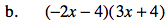Follow the steps in part (a).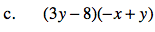Follow the steps in part (a).

−6x2 − 20x − 16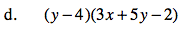Follow the steps in part (a).

3xy + 5y2 − 22y − 12x + 8